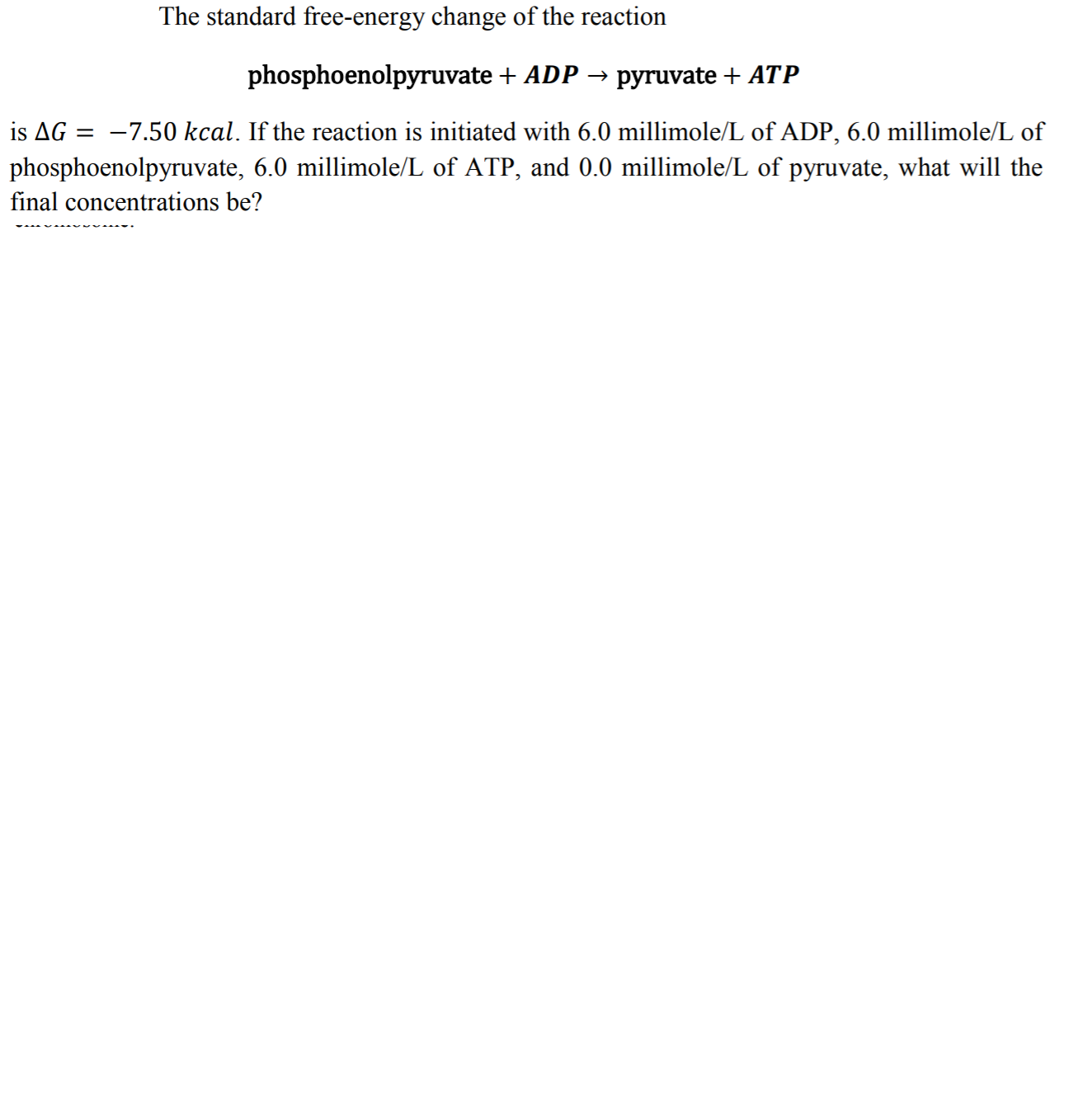# The standard free-energy change of the reactionphosphoenolpyruvate ADP-pyruvate + ATPis ΔG =-7.50 kcal. If the reaction is initiated with 6.0 millimole/L of ADP, 6.0 millimole/L ofphosphoenolpyruvate, 6.0 millimole/L of ATP, and 0.0 millimole/L of pyruvate, what will thefinal concentrations be?

Question
26 viewshelp_outlineImage TranscriptioncloseThe standard free-energy change of the reaction phosphoenolpyruvate ADP- pyruvate + ATP is ΔG =-7.50 kcal. If the reaction is initiated with 6.0 millimole/L of ADP, 6.0 millimole/L of phosphoenolpyruvate, 6.0 millimole/L of ATP, and 0.0 millimole/L of pyruvate, what will the final concentrations be? fullscreen
check_circle

Step 1

From the given value of change in Gibbs free energy of the process, the equilibrium constant can be calculated as:

Step 2

The value of R = 2 cal/Kmol

Since, the value of Gibbs free energy is standard, thus temperature = T = 25 0C = (25+273 ) K = 298 K

Thus, the equilibrium constant of the process can be calculated using equation (1):

Step 3

The ICE table for the given react...

### Want to see the full answer?

See Solution

#### Want to see this answer and more?

Solutions are written by subject experts who are available 24/7. Questions are typically answered within 1 hour.*

See Solution
*Response times may vary by subject and question.
Tagged in

### Chemistry